# C2000 Solar MPPT tutorial Pt/2

In this second part of the C2000 Solar MPPT Tutorial, the hardware and circuit design will be looked at in greater depth.  The schematic for the system is posted again below for reference, which can be enlarged by simply clicking on it.## Buck Converter Design

The first step was to design the buck circuit, this is determined by the output parameters of the system and it’s load.  For the first prototype based around the panel purchased for testing, it was decided to aim for a 12V output, therefore a maximum current of 750mA (assuming 10% losses).

When calculating a buck circuit the frequency of operation, inductor size and output capacitor size are important, as they determine the current and voltage ripple size.  It is desirable to have as smaller current and voltage ripple as possible.  A large current ripple can cause additional losses in a system, as there maybe times when the peak current is greater than the load requirements.  A large voltage ripple is obviously not desirable, good quality regulated power supplies have very low voltage ripples.

A general rule is the higher the frequency the smaller the inductor and output capacitor size, and a smaller inductor and capacitor size generally lowers the system cost.  However higher PWM frequencies decrease the system efficiency due to switching losses in the mosfets, so a trade off has to be reached which meets the design constraints of the end system.

For this system a PWM frequency of 15kHz was chosen, based on this, the solar panel and other design parameters for the buck circuit calculations can be performed.  First we can determine the system duty cycle at MPP, note the duty cycle will change to track the MPP with differing irradiance.  A figure of 90% was used for the buck converter efficiency,a typically buck converter efficiency is 90% or greater.Next an ideal current ripple can be determined, it is important to note that the below formulae used only determines a single phase current ripple.ΔIL is the inductor ripple current, and in this case a 30% figure was used for the multiplier. Now that the current ripple is know the inductor size can be calculated with the following equation.Using two 1.34mH inductors on each phase will ensure the inductor ripple current is effectively halved, knowing this an inductor ripple current of 90mA can be used to calculate the minimum output capacitor size.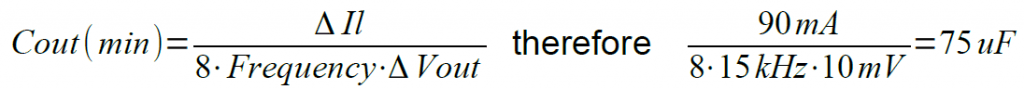Δvout is the desired ripple voltage.  The constant 8 is determined by the simplification of an equation, which can be found in various sources, one such source is an Application Note by On-Semiconductor AND9135/D.

There are two other factors for the inductor and capacitor that are important to consider: the inductor peak current and the capacitors Equivalent Series Resistance (ESR).  The inductor for this project will be hand wound using a toroidal core, which will be covered shortly.  The capacitors ESR can affect the reliability of the capacitor.  A capacitor will dissipate power as heat depending on it’s ESR, so a low ESR is desirable as excessive heating will shorten the life of a capacitor and be less efficient.  For this early prototype, cheap off the shelf capacitors were used as their reliability over time was not a concern at this stage.

The inductors were constructed using a T68-26A toroidal core, this core has a nominal inductance or Al value of 58nH.  The following equation was used to determine how many turns of wire the core would need.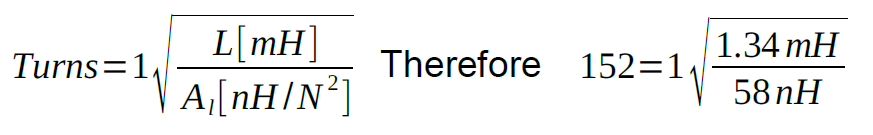A 0.3mm outside diameter enamelled cable was chosen, this has a maximum current rating of 1.4A.  Then a very useful website found here was used, this allows you to calculate the total length of cable required based on the toroidal core dimensions and cable diameter. Using a vice the cores were both wound and then measured using a LCR meter and measured at 1.3mH, an image below shows the hand winding process used.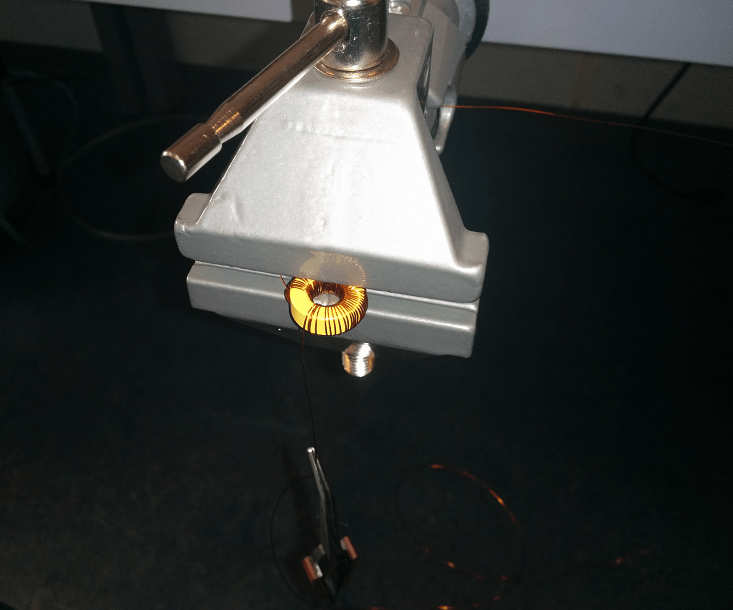## MOSFET Losses

For the prototype system Vishay IRFI640G MOSFETs were used, these are not the most efficient having a high RDSON value (180mΩ), but they were stocked at the time of writing.

The power losses from the High Side and Low Side MOSFETs are a combination of conduction and AC switching losses.  The conduction losses are a result of I²R losses inside the MOSFET when it is fully on, and the switching losses are the result of the MOSFET transitions from its on and off states.  Some example calculations will now be shown using data from the IRFI640G datasheet and various sources, on a synchronous MOSFET buck circuit efficiency.The first equation is for the High Side MOSFET and is based on the Vmp of the solar panel at maximum output current running through each interleaved phase.The next equation is for the Low Side MOSFET using the same current figure.The results from these two equations can be combined to work out the overall efficiency, the losses will also be multiplied by a factor of 2 as this is for a two phase interleaved circuit.Now if we increase the PWM frequency to 150kHz, the circuit losses will also be shown to increase.The switching losses in this next example will be shown to increase with increasing current, the next example uses a 15kHz switching frequency but with 2A, increasing the overall power to 24W.A MOSFET with a lower RDS(ON) will have lower conduction losses, but it will typically have a higher QG (Gate Charge) resulting in higher switching losses.  Therefore a careful balance between these characteristics should be found to maximise the circuits efficiency.  Taking into account the nominal parameters of the system, such as input voltage, output current, switching frequency and duty cycle, will allow the best efficiency to be achieved.  This will often involve using MOSFETs with different characteristics for the High and Low Side drivers.

## MOSFET Driver Circuit

The Half-Bridge (H-Bridge) driver is an IC designed specifically for driving MOSFETs. The IC takes the incoming PWM signal, and then drives two outputs for a High and a Low Side MOSFET.  This type of H-bridge is often used to to drive motors, but has other applications such as the following example.  The IC used is a IRS2003 half bridge driver made by International Rectifier, the image below shows an example circuit from the datasheet.The IRS2003 allows 2 PWM signals to be connected to HIN and LINNot this gives the user the opportunity to fine tune the dead-band switching of the MOSFETs.  The capacitor wired between VB and VS along with the diode form a charge pump, this allows the drive voltage to the MOSFETs to be almost doubled.  HIN and LINNot in this case are wired together and supplied with the same PWM signal, the IRS2003 has internal timing to ensure the High Side MOSFET, and the Low Side MOSFET are never on at the same time.  The capacitor between VB and VS needs to be sized to ensure it can drive enough current to the gate of the chosen MOSFET, over coming the gate capacitance.

There are four ADC ports used on this project, two sampling voltage and two sampling current.  The hardware employed to sample the voltage signals will be covered first, followed by the current sampling circuit.  But first a brief introduction to the TMS320F28027 ADC.

The ADC measures voltage from 0V to 3.3V, with a 12bit resolution.  It is important to not exceed the input voltage of the microcontrollers GPIO pins, the TMS320F28027 has a maximum input voltage of 3.63V.  Using this information the step resolution for the ADC can be calculated.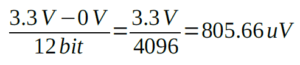The input on the ADC also has a small internal capacitance and resistance, this is used for Sample and Hold acquisition depending on the characteristics of the circuit being sampled. The internal ADC circuit taken from the TMS320x2802x datasheet is shown below.To ensure the readings being sampled are as accurate as possible, the source resistance or RS shown in the above image ideally needs to be as small as possible.  This will be achieved by placing an opamp configured as a unity gain buffer in all the ADC sample circuits.  The unity gain buffer will ensure a high input impedance, therefore reducing loading effects on the sampled circuitry to a minimum, as well as offering a very low output impedance to the C2000 internal ADC circuit.  Rail to rail opamps were used and supplied with 3.3V, this ensured the voltage passed to the ADC would not exceed this, thus ensuring the system has ADC protection built in.

The voltage sampling circuits consist of a simple potential divider, the maximum voltage the solar panel can produce is 21.6V when open circuit.The same resistor and opamp configuration is used for the input and output voltage measurement.  A more complex opamp circuit could have been used with offset, to fully exploit the range of the ADC, however this would provide more than enough accuracy for the prototype.

The current sampling circuit involved a slightly more complex approach.  The circuit would revolve around a Texas Instruments INA138 High Side Measurement current shunt monitor. The INA138 is basically a differential amplifier housed inside a small package, with a wide operating voltage.  The INA138 would be supplied with 12V, this then allows for a greater range to be measured around the 0V-3.3V range and was also the second supply voltage available for this circuit.  A typical configuration taken from the datasheet is shown in the image below.There are differences in input and output current so two formulas would be needed to ensure the range was correct.  The shunt resistors comprised of two 1Ω (1%) resistors in parallel, so the combined value becomes 0.5Ω.  The parallel resistors were measured and the actual value was approximately 0.47Ω.  A quick calculation to check these could withstand the power loads was made.This was not the best long term solution, but within tolerance for the 1% resistors in parallel.

The shunt resistor is connected directly across the internal differential amplifiers inputs. The calculations for the INA138 are fairly simple, the gain resistor soldered externally determines the overall gain of the device.  The datasheet for the INA138 states that the device has a gain of 1 with a 5kΩ resistor, gain of 2 with a 10kΩ, gain of 5 with a 25kΩ and so on and so forth. The input and output current gain resistors were calculated as follows.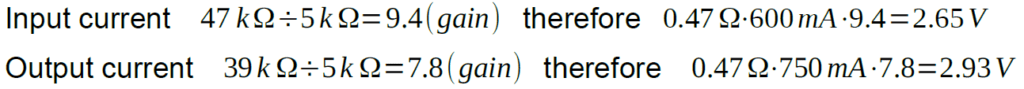These values would ensure the output from both the current feedback circuits falls in-line with the ADC input.

## Schematic Design and Layout

Certain aspects of the design were simulated in OrCad 16.6 first, to back-up the theory with simulation.  Then the design was taking over to EagleCad to enable faster prototyping.  Component symbols and foot prints where designed for all the non standard parts, ensuring these were all compatible with the LPKF milling machine used.  Once the schematic design was complete and the Electrical Rule Check (ERC) and Design Rule Check (DRC) were satisfactory, a schematic design was made for a two layer board.  The layout kept the PWM and digital switching side and analogue circuitry away from each other to avoid unnecessary noise.  The below image shows the final PCB prototype layout.The next part of this tutorial will go more in-depth into the C2000 software and Perturb and Observe algorithm, also including a downloadable version of the C code.

I take great care when writing all the tutorials and articles, ensuring all the code is fully tested to avoid issues for my readers.  All this takes time and a great deal of work, so please support the site by using the Adfly links etc.  If you have found this useful or have any problems implementing, please feel free to leave a comment and I will do my best to help.